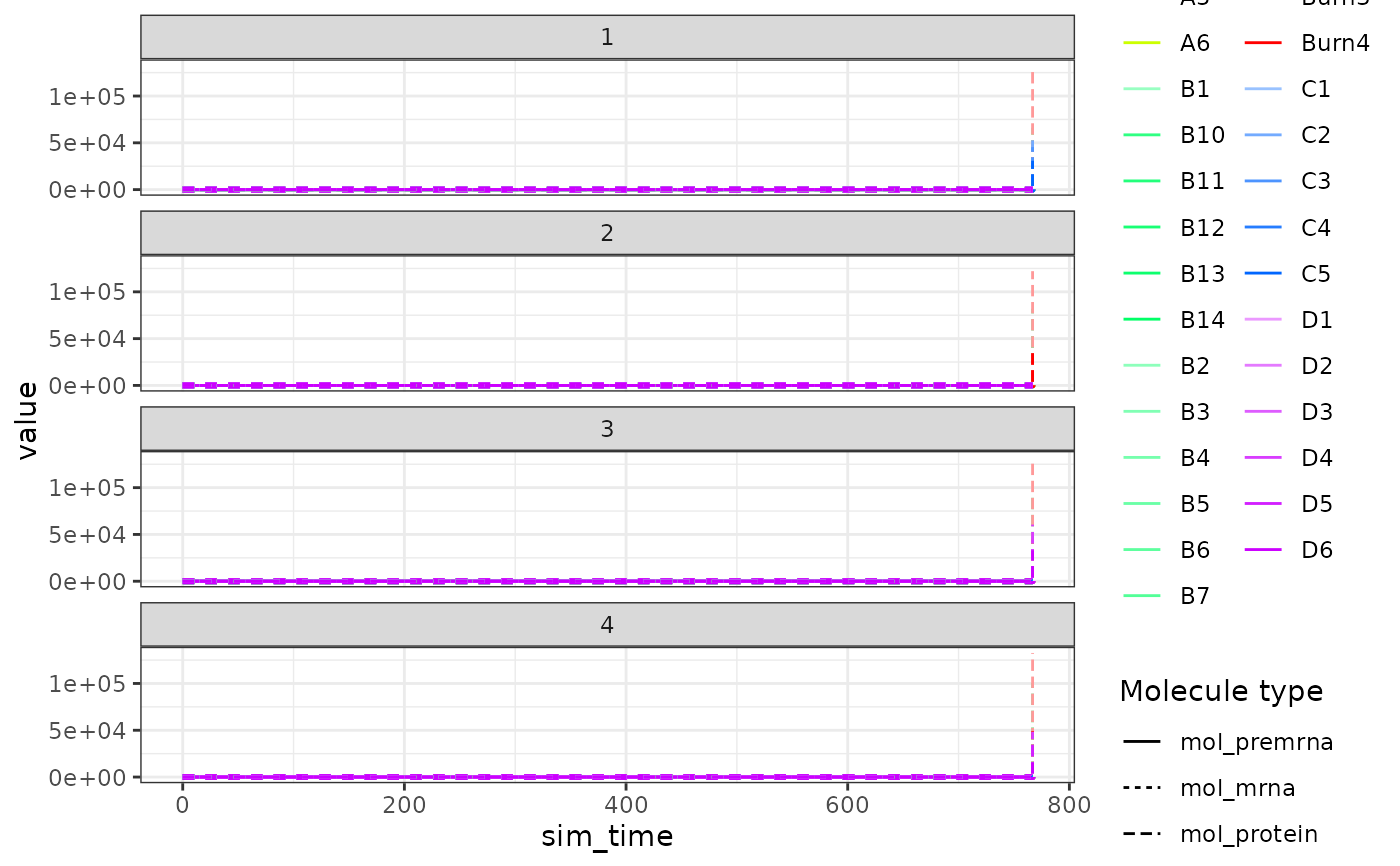Visualise the expression of the simulations over simulation time

plot_simulation_expression(
model,
simulation_i = 1:4,
what = c("mol_premrna", "mol_mrna", "mol_protein"),
facet = c("simulation", "module_group", "module_id", "none"),
label_nonzero = FALSE
)

## Arguments

model A dyngen intermediary model for which the simulations have been run with generate_cells(). Which simulation to visualise. Which molecule types to visualise. What to facet on. Plot labels for non-zero molecules.

## Value

A ggplot2 object.

## Examples

# \donttest{
data("example_model")
plot_simulation_expression(example_model)# }